# SS 2010 HKE Examination Microelectronics

## Problem 1

1.1: Identify the DRAM cell types shown in the schematic (2 points).
 ____ SRAM ____ DRAM ____ Flash1.2: Give the main differences between a SRAM, DRAM, and Flash memory cell (5 points)

## Problem 2

Given are the following design rules and a circuit layout.
1. Why are design rules needed? (2 points)
2. Draw the schematic of the layout below. Mark all design violations in the layout. (5 points)
3. What is the width and the length of the transistors. The smallest feature size in this process is 0.5 µm. (1 point)
 Design Rules Layout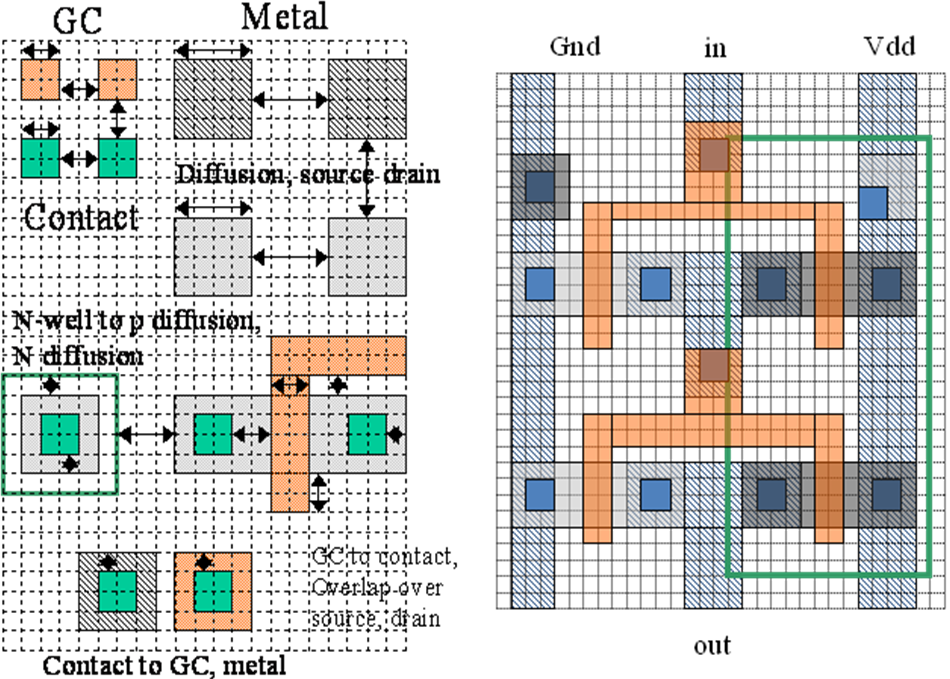## Problem 3

Label the different regions (Gate, Drain, Source, Isolation, Polysilicon, Metal) in the shown cross section of a transistor.
What type of transistor is it? (5 points)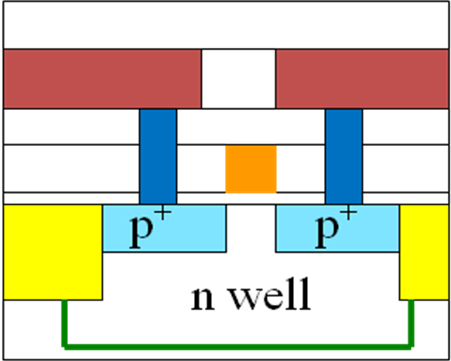## Problem 4:

1. Calculate the delay and rise and fall time of the output for a rising and falling output using the following properties. (6 points)
2. Calculate the input capacitance of the circuit. (2 points)
3. How would you optimize the circuit? (1 point)

 Technology Rn [Ω] Rp [Ω] Scale factor Cox=C'oxWL·(scale)2 1µm (long-channel) 15k L/W 45k L/W 1µm (1.75fF)·WL## Problem 5: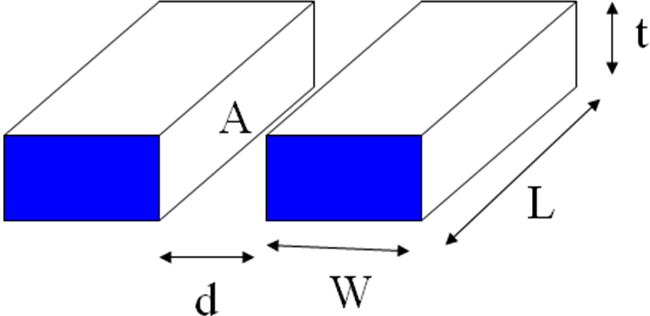d=1µm, t=0.5µm, W=2µm, Sheet Resistance Rsquare=100Ω/square, L=200µm
Plate Capacitance Cpl=30aF/µm2, Fringe Capacitance Cfr=80aF/µm
1. Calculate the resistance of a polysilicon line with length L. (2 points)
2. If the line resistance is too high, what can you do to lower the resistance? (2 points)
3. Calculate the capacitance between the 2 lines. (3 points)

## Problem 6:

Make a truth table for the following circuit.
Which Boolean function is it? (4 points)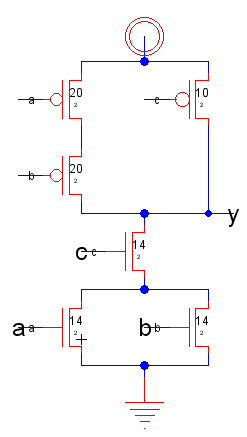A B C Y

## Problem 7:

Make a truth table and draw a schematic for the following logic AOI function. Size the transistors with minimum size to achieve a maximum delay like a inverter with PFET(15/1) and NFET(5/1).   (8 points)

$Z = \overline{ (AB)+C+D}$
 A B C D Z

## Problem 8:

1. Calculate the maximum number of chips with 150mm2 area which fit on a 300mm wafer. (3 points)
2. There is 1 defect per 600mm2. How many chips will fail? (1 point)
3. During test of all chips 38 chips are bad. What is the yield? What is the fault coverage? (4 points)
4. Is this a good fault coverage? (2 points)
5. What is the difference between a defect and a fault? (2 points)

# SS 2011 Microelectronics Problems

## Problem 1: Chip area and cost

A DRAM memory cell has a size of 8F2. 55% of chip area can be used for memory cells, 45% of chip area is used for periphery circuits and IO pads.
1. How big is a 1GBit chip in 70nm technology?
2. How many chips fit on a wafer with 200mm diameter?
3. How many good chips do you get with 70% yield?
4. What is the defect density for this yield?

## Problem 2: VHDL

 entity ThisCircuit is port ( INSIGNAL : in std_logic_vector (3 downto 0); OUTSIGNAL: out std_logic ); end ThisCircuit;   architecture ThisCircuit_BEHAVE of ThisCircuit is signal a: std_logic; begin  a <= INSIGNAL(2) or INSIGNAL(1) ;  OUTSIGNAL <= INSIGNAL(3) and a and INSIGNAL(0); end ThisCircuit_BEHAVE;
1. Draw a schematic described by this VHDL code.
2. Make a truth table of this circuit?
3. How many vectors do you need to test the circuit?

## Problem 3: Circuit and Layout Analysis

1. Calculate the input capacitance of the circuit on the right. The transistor has minimum length in 1µm technology.
 Technology Rn Rp Scale factor Cox=C'oxWL·(scale)2 1µm (long-channel) 15kL/W 15kL/W 1µm (1.75fF)·WL

2. Calculate the contact resistance of the drain and source contacts, if a single contact has a resistance of 10Ω.
3. A capacitance of 50pF is connected to the output. Calculate the propagation delays and rise and fall time of the output.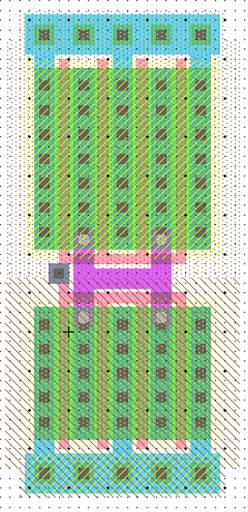## Problem 4: Truth table AOI circuit

 Make a truth table for the circuit on the right. How do you have to resize the transistors for equal delay to an inverter with a PFET W/L of 12/2 and NFET W/L of 6/2? Give a boolean equation for the circuit.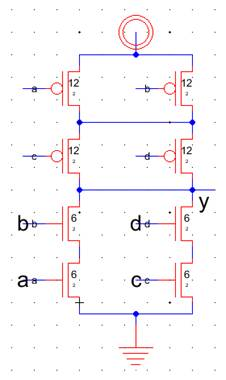## Problem 5: Circuit

Describe the input and outputs and the function of the circuit shown in the schematic. What kind of circuit is it?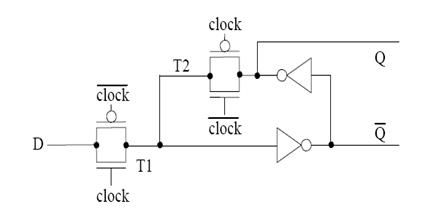## Problem 6: SPICE

This is the SPICE code of a netlist of a logic circuit with the input a and b and the output y.
*** TOP LEVEL CELL:
Mnmos@0 y a 0 0 N L=0.1U W=0.4U
Mnmos@1 y b 0 0 N L=0.1U W=0.4U
Mpmos@0 net@9 b y vdd P L=0.1U W=0.8U
Mpmos@1 vdd a net@9 vdd P L=0.1U W=0.8U
VD1 vdd 0 DC 1.2
Va a 0 PULSE(0 1.2 5n 1n 1n 4n 10n 20)
Vb b 0 PULSE(0 1.2 12.5n 1n 1n 9n 20n 20)
.include cmosedu_models.txt
.tran 0 25n 0 0.01n
.END

1. Draw a schematic of this circuit?
2. What is the operating voltage?
3. Draw a timing diagram of the signals a, b and y.
4. Why do you need the file cmosedu_models.txt?

# SS 2011 HKE Examination Microelectronics

## Problem 1:

A CPU with 50 Million Transistors needs 100F2 per transistor.

1. How big is the chip in 90nm technology? (2 points)
2. How many chips fit on a wafer with 300mm diameter? (3 points)
3. What defect density do you need to get a yield of 65%? (4 points).
4. How many more good chips do you get with the same defect density using a 70nm technology? (4 points).

## Problem 2:

Make a truth table for this VHDL code. (8 points)
 entity ThisCircuit is port ( op : in STD_LOGIC_VECTOR (1 downto 0); vin1,vin2 : in STD_LOGIC; vout : out STD_LOGIC); end ThisCircuit; architecture Behavioral of ThisCircuit is signal acc:STD_LOGIC; begin PROCESS (op) BEGIN CASE op IS WHEN "00" => acc<= '0'; WHEN "01" => acc<= vin1 or vin2; WHEN "10" => acc<= vin1 xor vin2; WHEN "11" => acc<= vin1 and vin2; WHEN OTHERS => acc<='0'; END CASE; END PROCESS; vout <= acc; end Behavioral;
Draw a timing diagram of the signals op, vin1, vin2 and vout of this circuit for the following stimulus. ( 6 points)
 constant Tp : time := 50 ns; stim_proc: process begin op <= "00"; vin1 <='0'; vin2 <='1'; wait for Tp; op <= "01"; vin1 <='1'; vin2 <='0'; wait for Tp; op <= "10"; vin1 <='0'; vin2 <='0'; wait for Tp; op <= "11"; vin1 <='1'; vin2 <='0'; wait for Tp; op <= "11"; vin1 <='1'; vin2 <='1'; wait for Tp; end process;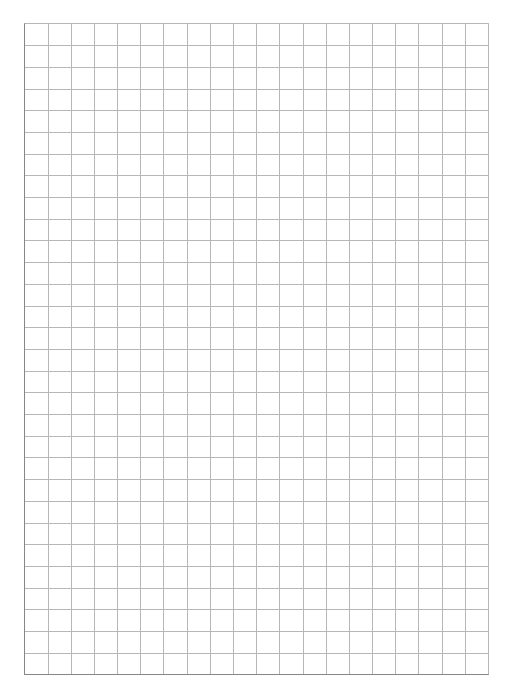## Problem 3: Test

1. Why do you have to test integrated circuits? (2 points)
2. Give an electrical model for a transition delay fault. (2 points)
3. Give an electrical model for a stuck at ´1 fault. (2 points)
4. What is a scan chain? What signals do you need and what is their purpose? How does a scan chain operate? What is the benefit and drawback of a scan chain? (12 points)

## Problem 4:

1. Calculate the minimum and maximum propagation delay for the circuit below, when a 10pF Capacitance is connected to the output. (4 points)
2. Calculate the maximum input capacitance of the circuit. (3 points)
3. What would be the W/L ratio of the transistors to optimize the circuit for minimum power consumption? Why is the power consumption lower? (4 points)
4. What would be the W/L ratio of the transistors to optimize the circuit to have a delay equal to an inverter with a NFET W/L 2/2 and PFET W/L 6/2? (4 points)

 Technology Rn Rp Scale factor Cox=C'oxWL·(scale)2 1µm (long-channel) 15kL/W 45kL/W 1µm (1.75fF)·WL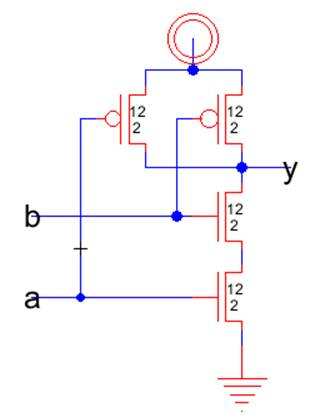## Problem 5:

For power supply routing 2 metal lines 2mm long and 30µm wide are running parallel to each other in 1µm distance over the chip. The lines are t=0.1µm thick. The metal sheet resistance is Rsquare=0.1Ω/square. The lines are isolated with oxide. (ε0= 88 fF/cm, εSiO2 = 4)1. Calculate the capacitance between the 2 metal lines.(2 points)
2. How could you change the capacitance between the lines in a given CMOS process? (5 points)
3. Calculate the voltage drop on the line if a current of 10µA is flowing. ( 2 points)

## Problem 6:

1. Make a truth table for the following circuit.(4 points)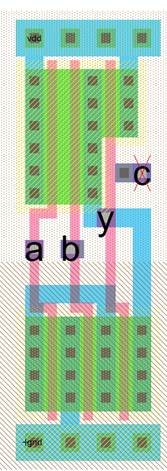A B C Y
2. Draw a transistor schematic.(4 points)
3. Give the boolean equation? (3 points)

## Problem 7: Microprocessor

1. What are the three states of a instruction decoder of a microprocessor? What happens during these states? (6 points)
2.  Describe step by step what happens with the registers IR, PC, ACC and Z during the execution of the program. (12 points)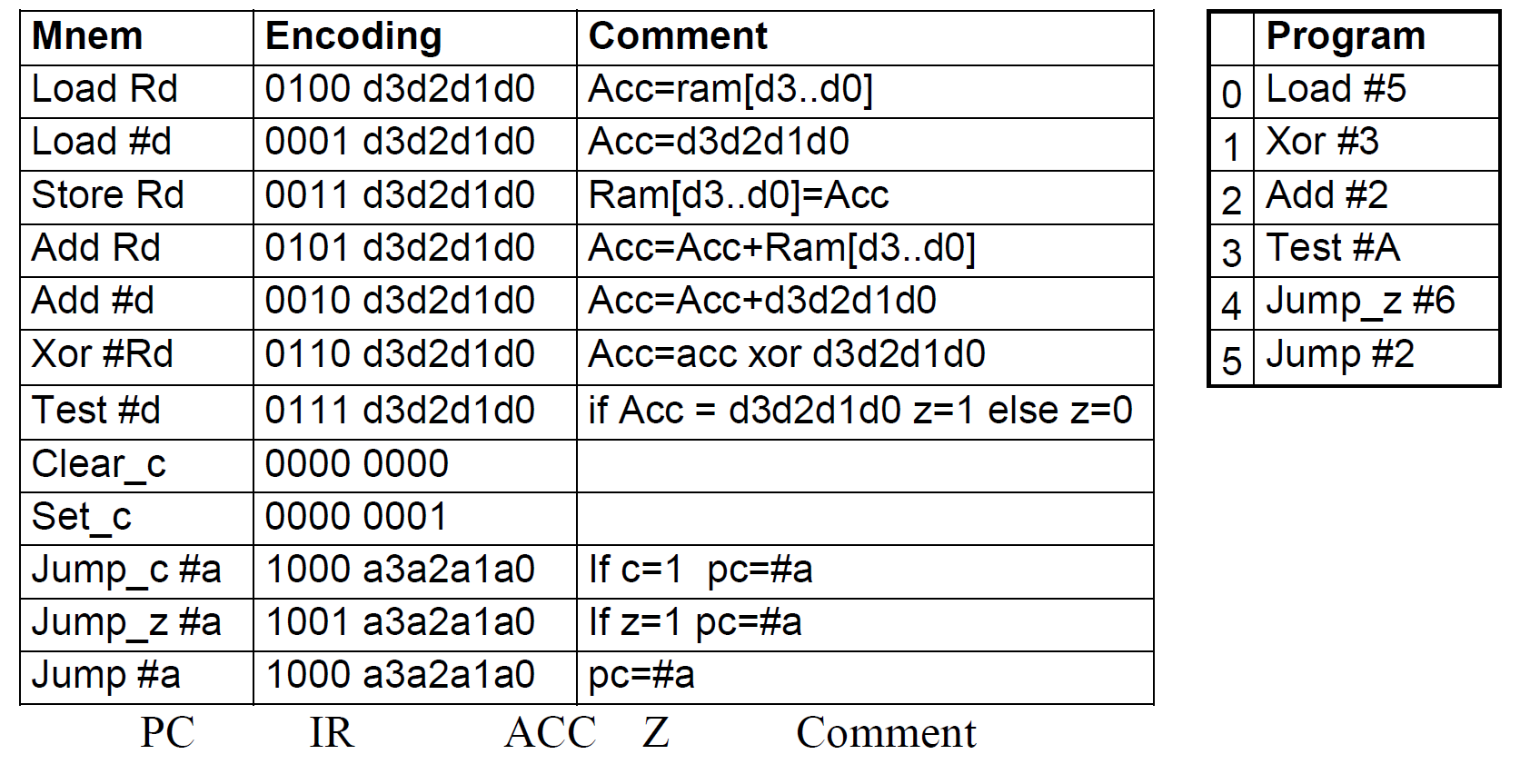## Problem 8: SPICE

*** SUBCIRCUIT Inv_1x FROM CELL Inv_1x{lay}
.SUBCKT Inv_1x VDD VSS Vin Vout
Mnmos@0 VSS Vin Vout gnd N_1u L=1U W=6U
Mpmos@1 VDD Vin Vout vdd P_1u L=1U W=12U
.ENDS Inv_1x
*** TOP LEVEL CELL: Test_dc_inv{lay}
XInv_1x@0 VDD 0 Vin Vout Inv_1x
XInv_1x@1 VDD 0 Vout Vres Inv_1x
*** SIGNALS
VIN Vin 0 DC 0
VDD_1 VDD 0 DC 2.9
.include cmosedu_models.txt
.dc VIN 0 3.3 10m
.END
1.  What simulation is done with this SPICE code? Describe the circuit, signals and simulation. (5 points)
2.  How many transistors are implemented, what are the dimensions and what is the transistor model name? (5 point)
3. What is the operating voltage? (1 point)
4.  Draw v(out),V(res) versus v(in) of this circuit. Label the axis and important measurement points. Discuss the curve. The PFET has Vtp=-0.8V and the NFET Vtn=0.8V. IDSN=2*IDSP. (11 points)

## Problem 9:

Make a truth table and draw a transistor and a logic schematic for the following logic AOI function. (15 points)

$Z = \overline{(AB) + (BCD)}$
 A B C D Z

# SS 2013 HKE Examination Microelectronics

## 1. Problem Manufacturing:

A wafer with 300mm diameter has 1600 Chips. During manufacturing test 110 chips have contact problems. 80 Chips violate the current limits. 180 Chips are functional fail. The chips are manufactured in a 60nm technology and need 80F2 per transistor.
1.1 What are the contact yield, the DC yield and the total yield? (6 points)
1.2 What is the defect density? (3 points)
1.3 How many transistors are realized on one chip? (2 points)
1.4 What would be the optimum width to length ratio of a chip? Why?(2 points)

## 2. Problem Circuit properties: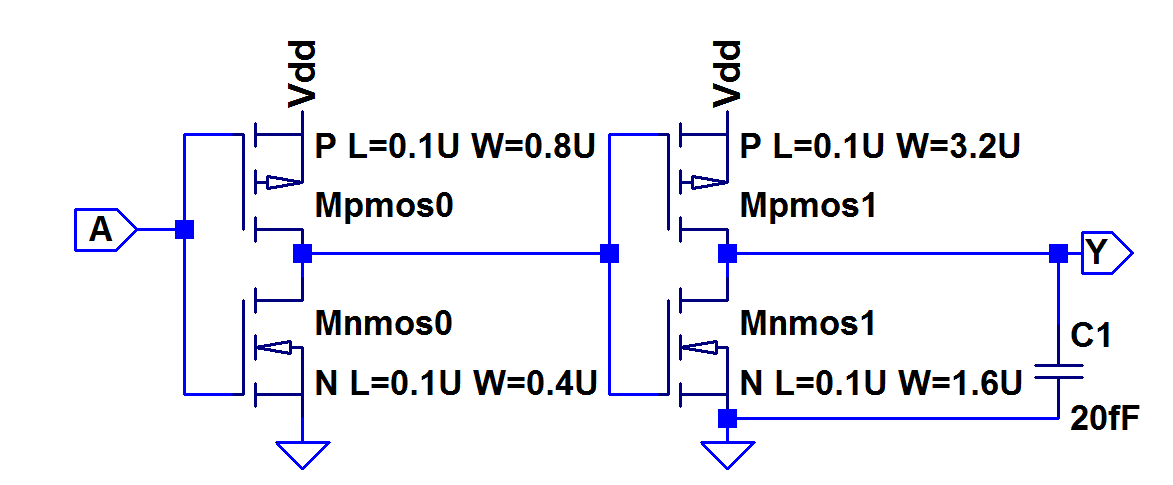Technology Scale factor Rn Rp Vthn Vthp Cox=Cox·WL(scale)2 50nm 34k Ω L/W 68k Ω L/W 280 mV 280 mV 62.5 aF·WL

The circuit is operated with VDD = 1V.
2.1. Calculate the minimum and maximum delay of this circuit.(6 points)
2.2 What is the maximum current of each of the 4 transistors?(5 points)

## 3. Problem Layout and parasitic:

 A top view of a metal line in a 50nm process running from A to B is shown crossing a polysilicon line, which runs from left to right. The metal sheet resistance is Rsquare=0.6Ω/square. The lines are isolated with 80nm oxide. (ε0= 88 fF/cm, εSiO2 = 4) 1. Calculate the capacitance between the metal line (blue) and the polysilicon line (yellow).(2 points) 2. Calculate the resistance from A to B? (2 points) 3. Calculate the voltage drop on the line if a current of 30µA is flowing. (2 points) 4. Draw a cross section from points C to D (4 points)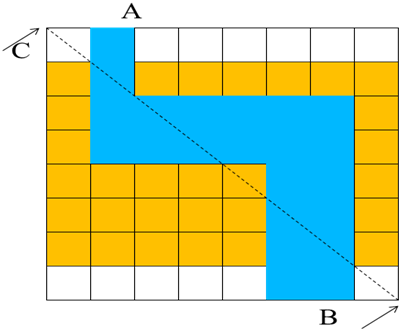## 4. Problem:

Make a truth table for the following circuit: (4 points)
 D A B C

Draw a transistor schematic with the W/L ratio of the transistors. (6 points)

Each contact has a resistance of 20Ω. What is the minimum and maximum contact resistance of the source drain contacts of the transistors? (3 points)

Draw a cross section along the line from A to B (5 points)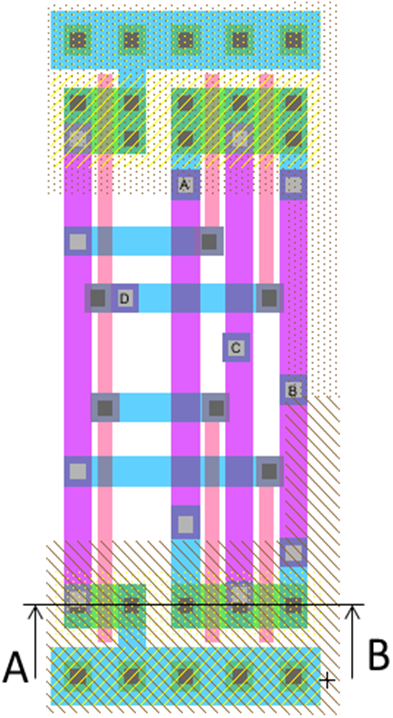## 5. Problem: Circuit Analysis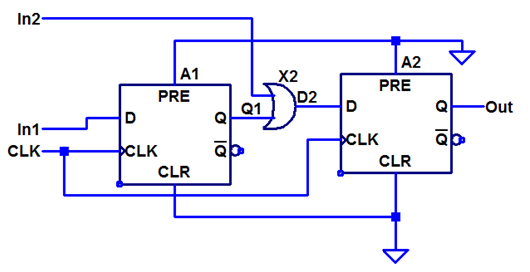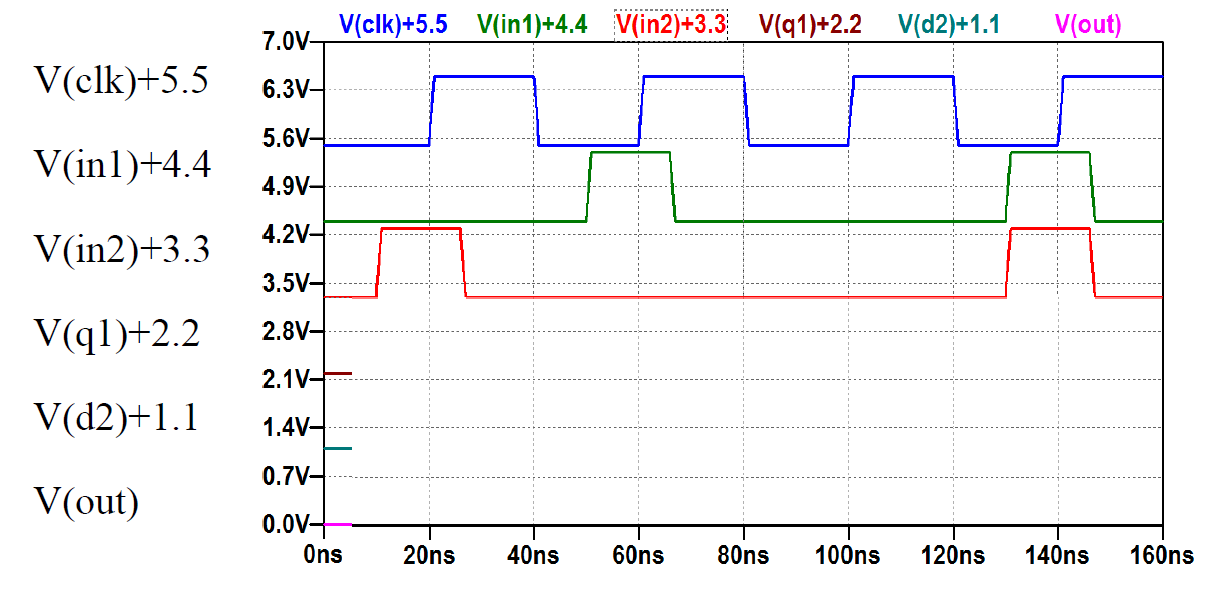5.1 Draw the output response of nodes Q1+2.2, D2+1.1 and Out. (6 points)
5.2 What are the setup and hold times? (6 points)

## 6. Problem: SPICE netlist

* Testcell
Mnmos0 N001 A 0 0 N L=0.1U W=0.4U
Mpmos0 N001 A Vdd Vdd P L=0.1U W=0.8U
Mnmos1 Y N001 0 0 N L=0.1U W=1.6U
Mpmos1 Y N001 Vdd Vdd P L=0.1U W=3.2U
C1 Y 0 100fF
VD1 vdd 0 DC 1.2
Va a 0 PULSE(0 1.2 5n 1n 1n 4n 10n 20)
.include cmosedu_models.txt
.tran 0 25n 0 0.01n

1. What simulation is done with this SPICE code? Make a schematic, describe the circuit, signals and simulation. (8 points)
2. How many transistors are implemented, what are the dimensions and what is the transistor model name? (5 points)
3. What is the operating voltage? (1 point)
4. Draw V(a), V(Y), V(N001) of this circuit for this simulation. Label the axis and important measurement points. Discuss the curve. (4 points)

## 7. Problem:

7.1 What is a silicon compiler? (2 points)
7.2 Why do you need DRC? (2 points)
7.3 What is alignment, what kind of alignment problems can happen, how is alignment done? (4 points)
7.4 What is specified with design rules? (3 points)
7.5 How do you make a robust layout? (3 points)

## Problem 8 VHDL:

1. Make a truth table for this VHDL code. (8 points)
 entity ThisCircuit1 is port ( clk: in STD_LOGIC; a: in STD_LOGIC_VECTOR (1 downto 0); b : in STD_LOGIC; y: out STD_LOGIC); end ThisCircuit1; architecture Behavioral of ThisCircuit1 is signal xy:STD_LOGIC; begin PROCESS (clk) BEGIN if (clk='1' and clk'event) then CASE a IS WHEN "00" => xy<=xy and b; WHEN "01" => xy<=a(0) or b; WHEN "10" => xy<=b and xy; WHEN "11" => xy<=a(1) and b; WHEN OTHERS => xy<='0'; END CASE; end if; END PROCESS; y <= xy or b; end Behavioral;

2. Draw a timing diagram of the signals a, b, c, xy and y of this circuit for the following stimulus. ( 7 points)
  constant Tp : time := 10 ns; BEGIN clk_process :process begin clk <= '0'; wait for Tp/2; clk <= '1'; wait for Tp/2; end process; stim_proc: process begin a <= "11"; b <='1'; wait for Tp; a <= "10"; b <='0'; wait for 2*Tp; a <= "00"; b <='1'; wait for Tp; a <= "01"; b <='0'; wait for Tp; a <= "11"; b <='0'; wait for Tp; end process;;# SS 2012 HKE Examination Microelectronics

## 1. Problem Manufacturing:

A wafer with 200mm diameter has 1200 Chips. During manufacturing test 20 chips have contact problems. 40 Chips violate the current limits. 80 Chips are functional fail. The chips are manufactured in a 90nm technology and need 100F2 per transistor.
1. What are the contact yield, the DC yield and the total yield? (6 points)
2. What is the defect density? (2 points)
3. How many transistors are realized on one chip? (2 points)
4. What can go wrong during manufacturing of integrated circuits? (6 points)

## 2. Problem Output voltage:

 Technology Scale factor Rn Rp Vthn Vthp Cox=Cox·WL(scale)2 50nm 34k Ω L/W 68k Ω L/W 280 mV 280 mV 62.5 aF·WL
All transistors have a width of 200nm and a length of 100nm.
1. Give the maximum voltages at the points OUT1, OUT2 and Out for an operating voltage of 1V. (4 points)
2. Calculate the delay from In1 to OUT1 through M1. (3 points)
3. Make a truth table. ( 4 points)

## 3. Problem layout and parasitic:

 A top view of a metal line in a 90 nm process running from A to B is shown crossing a polysilicon line, which runs from left to right. The metal sheet resistance is Rsquare = 0.4 Ω / square. The lines are isolated with 150 nm oxide. (ε0 = 88 fF/cm, εSiO2 = 4) Calculate the capacitance between metal line (blue) and the polysilicon line (yellow).(2 points) Calculate the resistance from A to B. (2 points) Draw a cross section from points C to D. (4 points)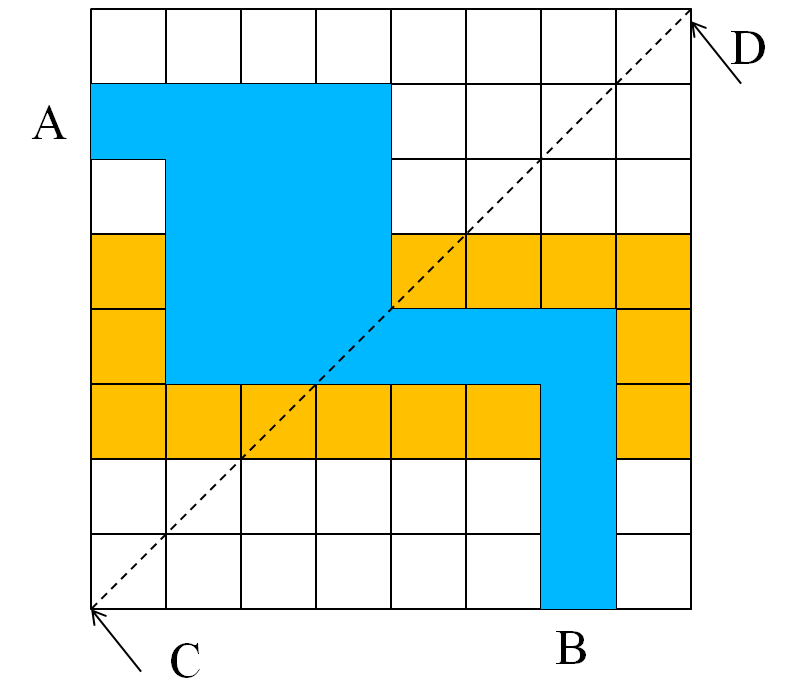## 4. Problem

Make a truth table for the following cicuit: (4 points)
 D C B A Y
Draw a transistor schematic with te W/L ratio of the transistors. (6 points)
Give the Boolean equation. (3 points)
Each contact has a resistance of 20 Ω. What is the minimum and maximum contact resistance of the source drain contacts of the transistors? (3 points)
Draw a cross section along the line from A to B (5 points)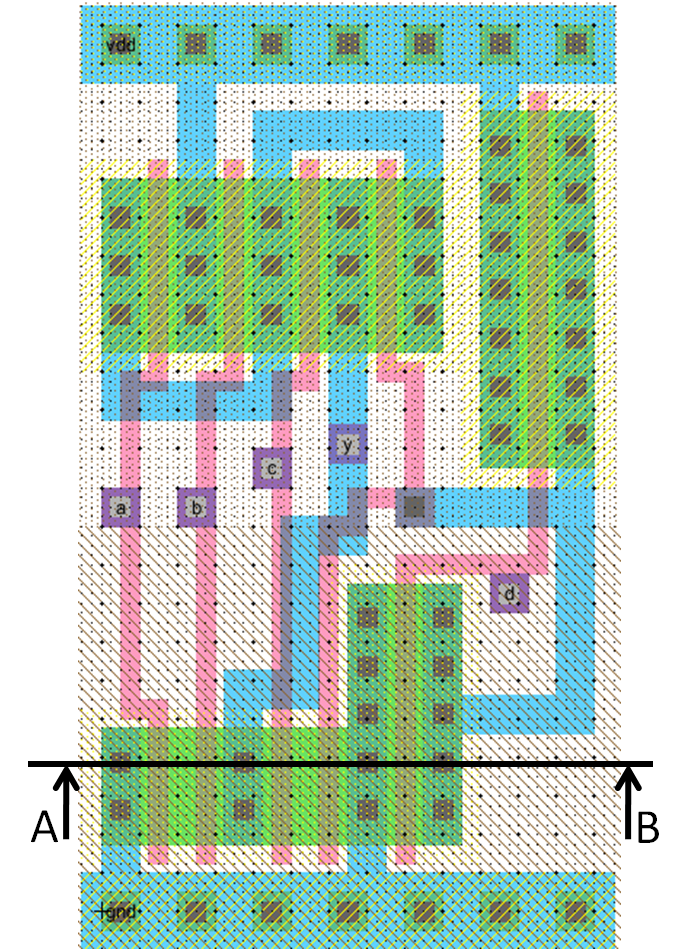## 5. Problem: Circuit analysis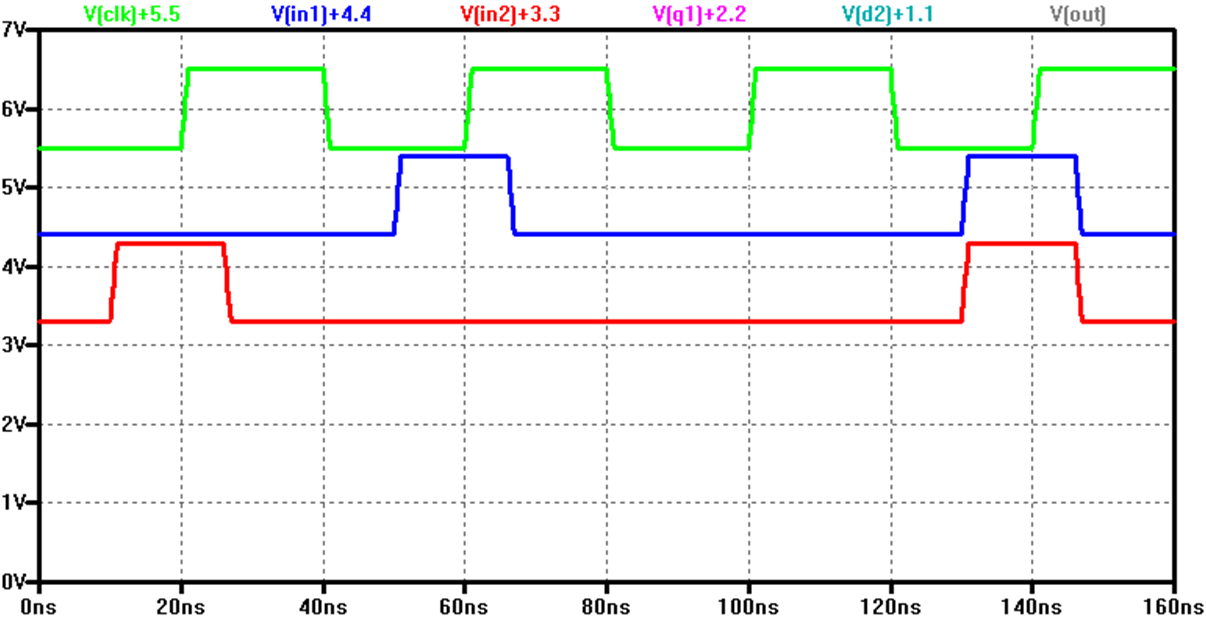Draw the output response of nodes Q1+2.2, D2+1.1 and Out. (6 points)
What are the setup and hold times? How do you measure a setup and hold time? (4 points)

## 6. Problem: SPICE netlist

* Testcell
M1 N001 N002 0 N003 NMx l=0.25u w=750n
R1 VDD N001 10k
C1 N001 0 20f
V1 N002 0 DC 0
V2 VDD 0 DC 2
V3 N003 0 DC 0
M2 N001 N002 0 N003 NMy l=0.5u w=750n
.dc V1 0 2 0.1 V3 0 -1 -1
.end

1. What simulation is done with this SPICE code? Make a schematic, describe the circuit, signals and simulation. (5 points)
2. How many transistors are implemented, what are the dimensions and what is the transistor model name? (5 point)
3. What is the operating voltage? (1 point)
4. Draw I(R1) versus V(V1) of this circuit for this simulation (Vthn0=0.5V). Label the axis and important measurement points. Discuss the curve. (5 points)

## 7. Problem

1. How do you cope with complexity? (4 points)
2. What is a floorplan? (2 points)
3. What are standard cells? What properties do they have? (4 points)
4. What is design verification? (2 points)
5. What is a wired AND fault model? (3 points)

## 8. Problem

(1) Make a truth table and draw (2) a transistor and (3) a logic schematic for the following AOI function. (15 points)
Z = (ABD) + (BC) + (AD)

# SS 2014 HKE Examination Microelectronics

## 1. Problem: Manufacturing

A wafer with 300mm diameter has 1200 Chips. The contact yield is 98% and there are 1035 functional pass chips.
1. What is the total yield and the relative functional yield? (2 points)
2. Calculate the defect density. (2 points)
3. What would be the total yield, if the chips would have half the area? (2 points)

## 2. Problem: Circuit properties

 Technology Scale factor Rn Rp Vthn Vthp Cox=Cox·WL(scale)2 50nm 34k Ω L/W 68k Ω L/W 280 mV 280 mV 62.5 aF·WL
The circuit is operated with VDD=1V.
1. Calculate the minimum and maximum delay of this circuit from A to Y.(8 points)
2. What is the minimum and maximum voltage of node B? (2 points)
3. What is the maximum current of Mpmos0? (2 points)

## 3. Problem: layout and parasitics

 A top view of a metal line in a 50nm process running from A to B is shown crossing a polysilicon line, which runs from left to right. The metal sheet resistance is Rsquare = 0.2 Ω/square. Plate capacitance is 40 aF/µm2 and fringe capacitance is 90 aF/µm. 1. Calculate the capacitance between the metal line (blue) and the polysilicon line (yellow).(3 points) 2. Calculate the resistance from A to B? (1 points) 3. Calculate the voltage drop on the line if a current of 30µA is flowing. (1 point) 4. Draw a cross section from points C to D (4 points)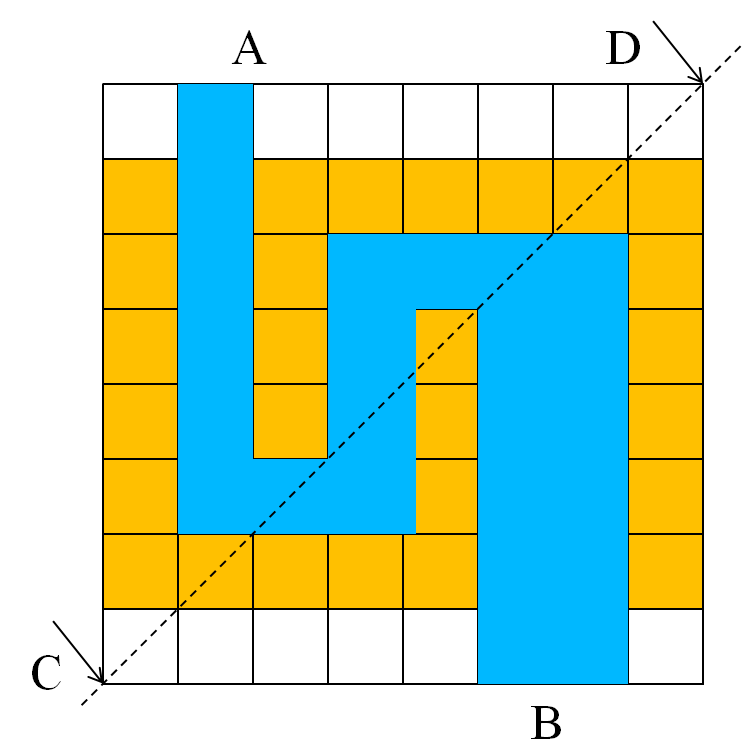## 4. Problem

Make a truth table for the following circuit: (4 points)
 C B A Y
Draw a transistor schematic with the estimated W/L ratio of the transistors. (6 points)
Each contact has a resistance of 20Ω. What is the minimum and maximum contact resistance of the source drain contacts of the transistors? (3 points)
Draw a cross section along the line from A to B (5 points)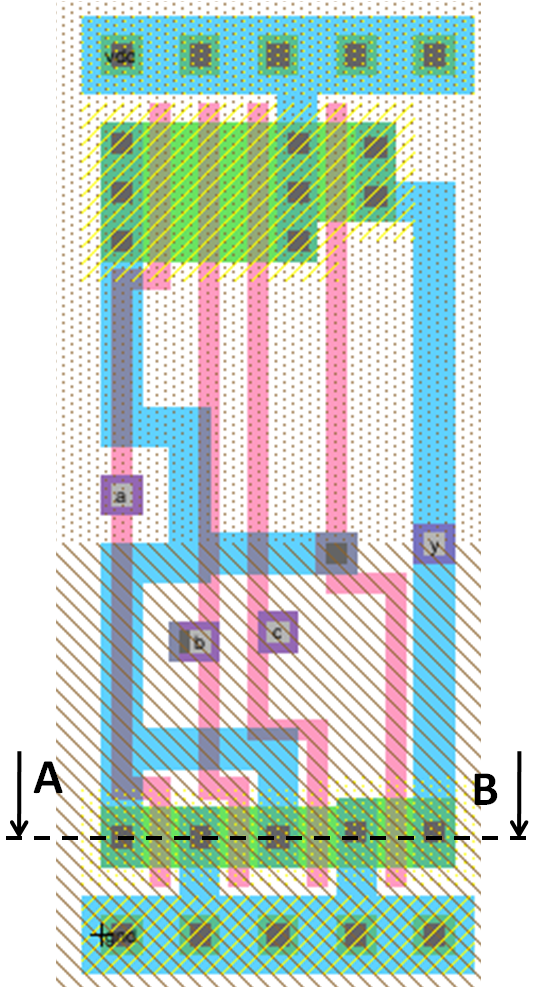## 5. Problem: Circuit Analysis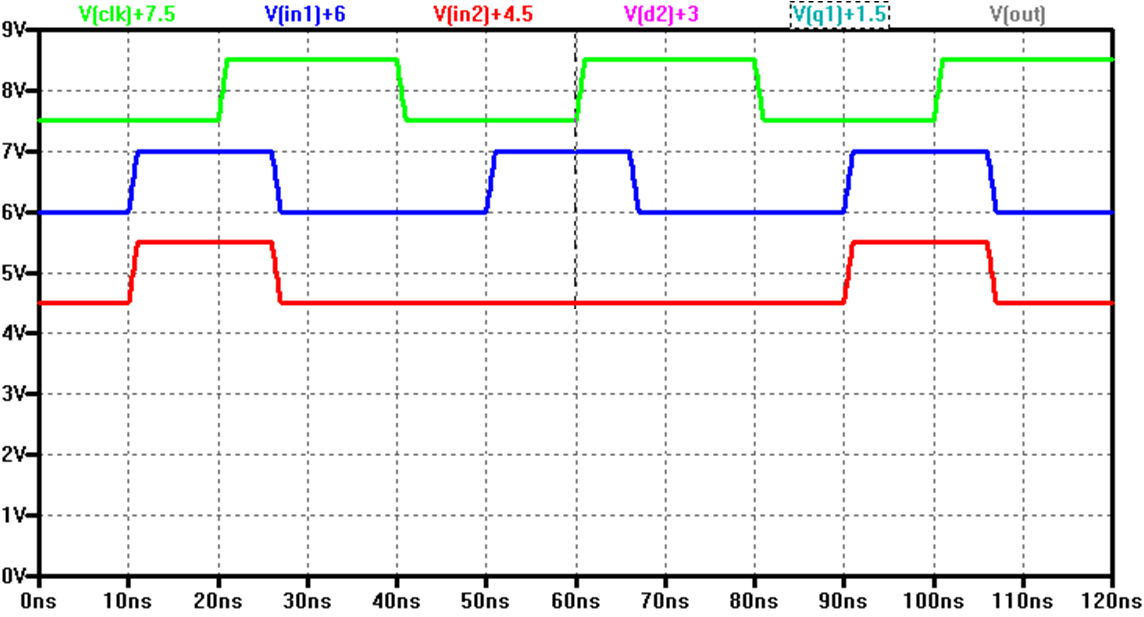5.1 Draw the output response of nodes d2+3.0, q1+1.5 and Out. (6 points)
5.2 What are the setup and hold times? (4 points)

## 6. Problem: SPICE netlist

* Testcell
Mnmos1 0 Y 0 0 NX L=0.1U W=2U
Mpmos1 Vdd Y Vdd Vdd PY L=0.1U W=3U
Mnmos0 Y Vdd A 0 NX L=0.5U W=4.0U
V1 A 0 SINE(0.5 0.5 50k) AC 1
VD1 vdd 0 DC 0.9
.include cmosedu_models.txt
.ac dec 10 100k 1G
.backanno
.end

1. What simulation is done with this SPICE code? Make a schematic, describe the circuit, signals and simulation. (8 points)
2. How many transistors are implemented, what are the dimensions and what is the transistor model name? (3 points)
3. What is the operating voltage? (1 point)
4. Draw V(a) and V(Y) of this circuit for this simulation. Label the axis and important measurement points. Discuss the curve. (4 points)

## 7. Problem

7.1 How can you control the gain of an amplifier? (2 points)
7.2 What is a stick diagram and why do you use it? (5 points)
7.3 What is a wired Or Fault model? (1 points)
7.4 What is electromigration and how do you prevent it? (4 points)

## 8. Problem: VHDL

Draw a schematic block diagram of the VHDL code. (8 points) Label the nets and instances.  entity Amp_test is port(Vin: in BIT; Vout: out BIT); end Amp_test; architecture Amp_test_BODY of Amp_test is component inv_1x1 port(a: in BIT; y: out BIT); end component; component tgr_1x1 port(x: in BIT; y: out BIT); end component; begin inv_1x1_0: inv_1x1 port map(net_0, Vout); tgr_1x1_1: tgr_1x1 port map(Vin, net_0); tgr_1x1_2: tgr_1x1 port map(Vout, gnd); tgr_1x1_3: tgr_1x1 port map(Vout, vdd); end Amp_test_BODY; `

## Questions:

1. What is a suitable unit for area per transistor?
2. Why are you interested in the area per transistor?
3. How can the switch point of an inverter be changed?
4. What is an RCX extraction? Why do you need it?
5. What is a process window investigation? Why do you need it?
6. What rules apply for a standard CMOS cell?
7. When do you do optimization?
8. How many delay times can be measured at a 3 input NOR gate?
9. How many test vectors do you need, if you have a circuit with 3 inputs and 4 registers?
10. A combinational circuit with 8 inputs is tested with 220 test vectors(input signal combinations). What is your test coverage?